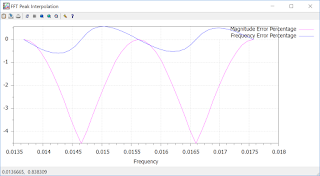## Saturday, 28 July 2018

### How To Interpolate The Output Of An FFT To Get Higher Resolution

One common requirement for a frequency analyzer is to maximize the amplitude or frequency estimation estimate for a given sinusoid.

One easy way to do this is to increase the size of the FFT however this comes at the cost of reduced time domain resolution and increased requirement for MIPS and memory.

A low impact solution to increase the resolution of the output is to interpolate the vertex to give a better estimate of the amplitude and frequency of an individual sinusoid.

The overall procedure is shown in the following diagram :FFT Interpolation Functions

Following the FFT function is a peak detector followed by a peak interpolator.
The interpolation function uses 3 point vertex quadratic interpolation, as shown in the following diagram.Peak Interpoation

Given that xm is the location of the peak and ym is the magnitude of the peak we can interpolate the peak value efficiently by setting xm-1 == 0, xm == 1, xm+1 == 2, which allows the interpolation to be implemented using the following equations :

a = (ym-1 - ym) * (ym-1 - ym+1)
b = -2 * (ym-1 -ym) + 0.5 * (ym-1 - ym+1)

Interpolated Frequency = -0.5 * b / a
Interpolated Magnitude = ym-1 - 0.25 * b2 / a

To analyze the results, we ran a series of sinusoids through the algorithm and plotted the percentage error. In the following graph we have a normalized sample rate of 1.0 Hz and we are using a 512 point FFT. Plotting the error percentage results between FFT bin 7 (0.013671875 Hz) and FFT bin 9 (0.017578125 Hz).Interpolation Function Results

We can observe that the maximum gain error percentage = 4.519154% and the maximum frequency error percentage = 0.596755%.

Note, this solution is very accurate when the signal constitutes a single sinusoid or a sinusoid sampled with low noise. However the accuracy will vary with increasing noise an/or adjacent sinusoids - chosing a good windowing function will definitely help with the latter.

Functions to implement the FFT interpoator are included in the SigLib DSP library. To evaluate the Numerix-DSP Libraries : http://www.numerix-dsp.com/eval/.